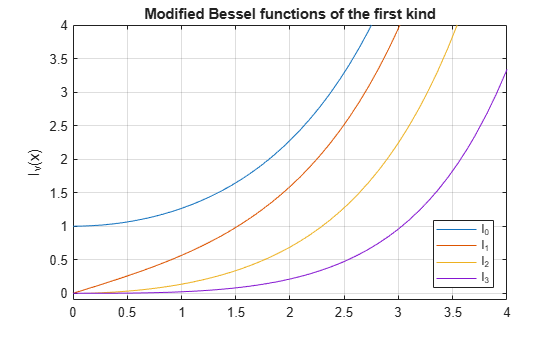# besseli

Modified Bessel function of the first kind for symbolic expressions

## Syntax

``besseli(nu,z)``

## Description

example

````besseli(nu,z)` returns the modified Bessel function of the first kind, Iν(z).```

## Examples

### Find Modified Bessel Function of First Kind

Compute the modified Bessel functions of the first kind for these numbers. Because these numbers are not symbolic objects, you get floating-point results.

`[besseli(0, 5), besseli(-1, 2), besseli(1/3, 7/4), besseli(1, 3/2 + 2*i)]`
```ans = 27.2399 + 0.0000i 1.5906 + 0.0000i 1.7951 + 0.0000i -0.1523 + 1.0992i```

Compute the modified Bessel functions of the first kind for the numbers converted to symbolic objects. For most symbolic (exact) numbers, `besseli` returns unresolved symbolic calls.

```[besseli(sym(0), 5), besseli(sym(-1), 2),... besseli(1/3, sym(7/4)), besseli(sym(1), 3/2 + 2*i)]```
```ans = [ besseli(0, 5), besseli(1, 2), besseli(1/3, 7/4), besseli(1, 3/2 + 2i)]```

For symbolic variables and expressions, `besseli` also returns unresolved symbolic calls:

```syms x y [besseli(x, y), besseli(1, x^2), besseli(2, x - y), besseli(x^2, x*y)]```
```ans = [ besseli(x, y), besseli(1, x^2), besseli(2, x - y), besseli(x^2, x*y)]```

### Solve Bessel Differential Equation for Modified Bessel Functions

Solve this second-order differential equation. The solutions are the modified Bessel functions of the first and the second kind.

```syms nu w(z) dsolve(z^2*diff(w, 2) + z*diff(w) -(z^2 + nu^2)*w == 0)```
```ans = C2*besseli(nu, z) + C3*besselk(nu, z)```

Verify that the modified Bessel function of the first kind is a valid solution of the modified Bessel differential equation.

```syms nu z isAlways(z^2*diff(besseli(nu, z), z, 2) + z*diff(besseli(nu, z), z)... - (z^2 + nu^2)*besseli(nu, z) == 0)```
```ans = logical 1```

### Special Values of Modified Bessel Function of First Kind

If the first parameter is an odd integer multiplied by 1/2, `besseli` rewrites the Bessel functions in terms of elementary functions:

```syms x besseli(1/2, x)```
```ans = (2^(1/2)*sinh(x))/(x^(1/2)*pi^(1/2))```
`besseli(-1/2, x)`
```ans = (2^(1/2)*cosh(x))/(x^(1/2)*pi^(1/2))```
`besseli(-3/2, x)`
```ans = (2^(1/2)*(sinh(x) - cosh(x)/x))/(x^(1/2)*pi^(1/2))```
`besseli(5/2, x)`
```ans = -(2^(1/2)*((3*cosh(x))/x - sinh(x)*(3/x^2 + 1)))/(x^(1/2)*pi^(1/2))```

### Differentiate Modified Bessel Function of First Kind

Differentiate the expressions involving the modified Bessel functions of the first kind:

```syms x y diff(besseli(1, x)) diff(diff(besseli(0, x^2 + x*y -y^2), x), y)```
```ans = besseli(0, x) - besseli(1, x)/x ans = besseli(1, x^2 + x*y - y^2) +... (2*x + y)*(besseli(0, x^2 + x*y - y^2)*(x - 2*y) -... (besseli(1, x^2 + x*y - y^2)*(x - 2*y))/(x^2 + x*y - y^2)) ```

### Bessel Function for Matrix Input

Call `besseli` for the matrix `A` and the value 1/2. The result is a matrix of the modified Bessel functions ```besseli(1/2, A(i,j))```.

```syms x A = [-1, pi; x, 0]; besseli(1/2, A)```
```ans = [ (2^(1/2)*sinh(1)*1i)/pi^(1/2), (2^(1/2)*sinh(pi))/pi] [ (2^(1/2)*sinh(x))/(x^(1/2)*pi^(1/2)), 0]```

### Plot the Modified Bessel Functions of the First Kind

Plot the modified Bessel functions of the first kind for $v=0,1,2,3$.

```syms x y fplot(besseli(0:3, x)) axis([0 4 -0.1 4]) grid on ylabel('I_v(x)') legend('I_0','I_1','I_2','I_3', 'Location','Best') title('Modified Bessel functions of the first kind')```## Input Arguments

collapse all

Input, specified as a number, vector, matrix, array, or a symbolic number, variable, expression, function, or array. If `nu` is a vector or matrix, `besseli` returns the modified Bessel function of the first kind for each element of `nu`.

Input, specified as a number, vector, matrix, array, or a symbolic number, variable, expression, function, or array. If `nu` is a vector or matrix, `besseli` returns the modified Bessel function of the first kind for each element of `nu`.

collapse all

### Modified Bessel Functions of the First Kind

The modified Bessel differential equation

`${z}^{2}\frac{{d}^{2}w}{d{z}^{2}}+z\frac{dw}{dz}-\left({z}^{2}+{\nu }^{2}\right)w=0$`

has two linearly independent solutions. These solutions are represented by the modified Bessel functions of the first kind, Iν(z), and the modified Bessel functions of the second kind, Kν(z):

`$w\left(z\right)={C}_{1}{I}_{\nu }\left(z\right)+{C}_{2}{K}_{\nu }\left(z\right)$`

This formula is the integral representation of the modified Bessel functions of the first kind:

`${I}_{\nu }\left(z\right)=\frac{{\left(z/2\right)}^{\nu }}{\sqrt{\pi }\Gamma \left(\nu +1/2\right)}\underset{0}{\overset{\pi }{\int }}{e}^{z\mathrm{cos}\left(t\right)}\mathrm{sin}{\left(t\right)}^{2\nu }dt$`

## Tips

• Calling `besseli` for a number that is not a symbolic object invokes the MATLAB® `besseli` function.

• At least one input argument must be a scalar or both arguments must be vectors or matrices of the same size. If one input argument is a scalar and the other one is a vector or a matrix, `besseli(nu,z)` expands the scalar into a vector or matrix of the same size as the other argument with all elements equal to that scalar.

 Olver, F. W. J. “Bessel Functions of Integer Order.” Handbook of Mathematical Functions with Formulas, Graphs, and Mathematical Tables. (M. Abramowitz and I. A. Stegun, eds.). New York: Dover, 1972.

 Antosiewicz, H. A. “Bessel Functions of Fractional Order.” Handbook of Mathematical Functions with Formulas, Graphs, and Mathematical Tables. (M. Abramowitz and I. A. Stegun, eds.). New York: Dover, 1972.

#### Mathematical Modeling with Symbolic Math Toolbox

Get examples and videos